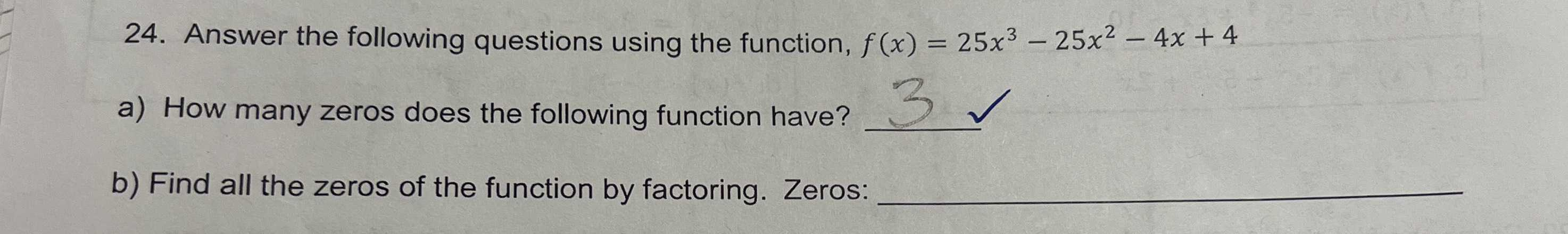### ¿Todavía tienes preguntas de matemáticas?

Pregunte a nuestros tutores expertos
Algebra
Pregunta24. Answer the following questions using the function, $$f ( x ) = 25 x ^ { 3 } - 25 x ^ { 2 } - 4 x + 4$$

a) How many zeros does the following function have?

b) Find all the zeros of the function by factoring. Zeros:

25x$$^{2}$$(x-1)-4(x-1)
(x-1)(25x$$^{2}$$-4)
x=1,$$\frac{2}{5},- \frac{2}{5}$$